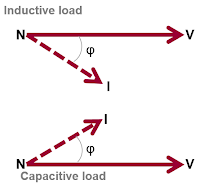## You need to test, we're here to help.

You need to test, we're here to help.

## 09 March 2018

### Power Calculations for Pure Sine WavesFigure 1: For a purely resistive load, power = voltage * current, with both vectors in phase
Wouldn't it be wonderful if every sine wave we encountered in the real world was pure, with no distortion? It sure would make life easier. Alas, it's pretty much never the case. But in reviewing sinusoidal power calculations, it's best that we begin with the simplest case: a single, pure sinusoidal line voltage and single, pure sinusoidal line current supplying a linear load.

Electric power is the rate at which energy is transferred to a circuit, calculated in units of Watts, with a Watt being an energy-transfer rate of 1 Joule/s. For a purely resistive load fed by a single current vector and single voltage vector,

P = I2R = V2/R = instantaneous V*I

with both vectors perfectly in phase (Figure 1).Figure 2: Phase angle is the angular difference between the voltage and current vectors
However, a purely resistive load is about as rare in the wild as the pure sine wave. There's typically some amount of inductance or capacitance. Depending on which of those is the dominant characteristic, the current vector will either lead or lag the voltage vector, and the quantity of lag or lead is defined by the phase angle (φ). Phase angle indicates the angular difference between the voltage and current vectors. It can be expressed in degrees (from -90° to +90°), or in radians (from -π/2 to +π/2).

The cosine of the phase angle is known as the "power factor" (PF or λ). Remember that PF = Cos(φ) only for purely sinusoidal waveforms. Power factor is always present in AC systems. It's a unit-less quantity ranging from 0 to 1. For a purely resistive load with V and I in phase, PF = 1. For a purely capacitive or a purely inductive load with V and I 90° out of phase, PF = 0. Power factor is typically not signed: current either leads (capacitive load) or lags (inductive load) voltage (Figure 2).Figure 3: Phase angle can be directly measured between a pure voltage sine wave and a pure current sine wave
Here's another way in which to think about phase angle. Figure 3 depicts a pure sinusoidal voltage and a pure sinusoidal current. Phase angle can be directly measured between these waveforms. We can see that the current has a different magnitude than the voltage.

At the zero crossing, we can also see that there's a time delay between the waveforms that can be expressed in degrees relative to a full period of 360°. That's the phase angle, and again, if these current and voltage waveforms were feeding a purely resistive load, they would be perfectly in phase and would be directly on top of each other. In this case, current leads voltage, indicating a capacitive load. Thus, for a single-phase, non-resistive load (meaning that it's either capacitive or inductive), P ≠ V*I, and we must account for the phase angle.

#### Three Types of Power

To break things down further, there are three different types of power:

• Apparent power (S), which is expressed in Volt-Amperes (VA) and calculated by multiplying the RMS voltage and current at any given point in time for a given power cycle. This assumes that we can measure "true RMS" values.
• Real power (P), which is expressed in Watts and derived from the apparent power by multiplying apparent power times the power factor. This assumes two sinusoidal waveforms; this derivation is never true if that's not the case.
• Reactive power (Q), which is expressed in Volt-Amperes reactive (VAr) and is the quadrature subtraction of the real from the apparent power:
Q = √(S2-P2)

Reactive power is not consumed, which is to say it's not transferred to the load during a power cycle, but rather simply circulates in the circuit during operation.

So to conclude, AC line voltages are very different from the 5- or 3.3-V logic voltages familiar to many engineers. Three-phase systems are a complex mix of magnitude, phase, and rotation, and they introduce different measurement challenges, such as the lag/lead of current vectors relative to voltage vectors. That results in more than one type of power (real, apparent, and reactive), so line "power" isn't a simple P = V*I situation.

Next time, we'll look at power calculations for distorted waveforms.

Previous posts in this series:

Back to Basics: The Fundamentals of Power
Back to Basics: Fundamentals of AC Line Power (Part II)
Back to Basics: Three-Phase Sinusoidal Voltages
More Basics of Three-Phase Sinusoidal Voltages
Back to Basics: AC Sinusoidal Line Current